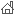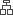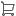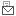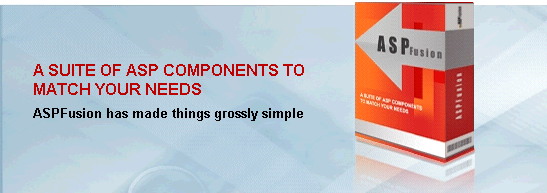AdvSMTPAdvIMAP4AdvPOP3AdvFileAdvZIPAdvGraphGeneratorAdvLDAPAdvHTTPAdvDSNAdvDirectoryAdvRegistryAdvMX
ASP Components >> AdvGraphGenerator
see also CF_GRAPH
Download | Buy
AdvGraphGenerator

The component allows you to create different types of analytical graphs against the given data, and to save it as an image file to enhance usability. The component provides these operations.Different types of graphs including
 a Bar graph b Line graph c Pie graph d Stacked bar graph e Box-Whisker graph f 3D bar graph g 3D line graph h 3D pie graph i 3D stacked bar graphDifferent categories of graphs including
 a Non series graphs (for a single set of analytical data) b Series graphs (for multiple set of analytical data)Customized graph options like, grid lines, labels, back-ground color, image height, image width, etc.Save graph in multiple formats, current supported formats are BMP and JPEG.

ASPFusion also provides ColdFusion custom tag CF_GRAPH written on AdvGraphGenerator component. This helps coldfusion developers in making a better understanding, in case they are using AdvGraphGenerator component.

Sample code for C++, ASP and CF is provided with the component.

Example (ASP):

<% set Obj = Server.CreateObject("AdvGraphGenerator.GraphGenerator") %>

Example (ColdFusion):

<CFOBJECT Class="AdvGraphGenerator.GraphGenerator" Action="Create" Name="Obj">

### AdvGraphGenerator Properties

 Property Description ChartTitle This property is used to give a title to the graph. Example (ASP): <% Obj.ChartTitle = "Monthly Salary Graph" %> Example (ColdFusion): ErrorReason Reports any error that occurred during the request. Example (ASP): <% Response.Write Obj.ErrorReason %> Example (ColdFusion): #Obj.ErrorReason# FileName This property is the name of the image file created. The image name must be followed by its extension. Extension can be only be JPEG or BMP. The file is saved with this name in the path set using property FilePah. If this name is missed the image will not be saved. Example (ASP): <% Obj.FileName = "barGraph.jpg" %> OR <% Obj.FileName = "barGraph.bmp" %> Example (ColdFusion): FilePath The full path of the directory or folder where the created image is to be saved. This property must be set before making any graph. If not set or the path is not valid then the image will not be saved. Example (ASP): <% Obj.FilePath = "C:\myGraphs" %> Example (ColdFusion): GraphHeight The property is used to set the height of the graph in pixels. This is optional to set, if not set then the default value is used i.e. 350. A value less then the 200 is not recommended as it may not display the scale on the Y-Axis properly. Example (ASP): <% Obj.GraphHeight = 425 %> Example (ColdFusion): GraphWidth The property is used to set the width of the graph in pixels. This is optional to set, if not set then the default value is used i.e. 500. A value less then the 300 is not recommended as it may not display the scale on the X-Axis properly. Example (ASP): <% Obj.GraphWidth = 600 %> Example (ColdFusion): SeriesLabel In case of multiple series graph this property is used as title for each series. Example (ASP): <%  Obj.SeriesLabel = "Series1" %> Example (ColdFusion): XAxisLabel The property is used to set a label to appear on the X-Axis. This property can be used to represent unit of measurement on the X-Axis. Example (ASP): <% Obj.XAxisLabel = "Months" %> Example (ColdFusion): YAxisLabel The property is used to set a label to appear on the Y-Axis. This property can be used to represent unit of measurement on the Y-Axis. Example (ASP): <% Obj.YAxisLabel = "Salary" %> Example (ColdFusion):

### AdvGraphGenerator Methods

 Method Parameter Return Value Description AddData 1. X-Axis Value 2. Y-Axis Value None This method is used to add data in the form of (X, Y) value pairs. This is the data that is used to generate graph. Note that first argument is STRING where as the second argument is NUMERIC. The second  argument is used as analytical data to generate graphs whereas the first argument is used to represent each corresponding Y-Axis values. Example (ASP): <% Obj.AddData  "Jan", 2000        Obj.AddData  "Feb", 4000        Obj.AddData  "Mar", 2500        Obj.AddData  "Apr", 1000        Obj.AddData  "May", 3000        Obj.AddData  "Jun", 1500 %> Example (ColdFusion): AddDataEx 1. Analytical Data None This method is used to add data that is used in creation of Series Graphs. This method varies from the first AddData method as it takes only one argument since in the series graphs, names of the elements are added prior to adding data. Note that AddDataEx must be called successively for each series data in the order the item names have been added using AddItemToSeries method. Example (ASP): <% Obj.AddItemToSeries "First Item"        Obj.AddItemToSeries "Second Item"        Obj.AddItemToSeries "Third Item"              Obj.AddSeries "Series1"               Obj.AddDataEx 20        Obj.AddDataEx 40        Obj.AddDataEx 25               Obj.AddSeries "Series2"               Obj.AddDataEx 10        Obj.AddDataEx 30        Obj.AddDataEx 15               Obj.DrawBarSeriesGraph() %> Example (ColdFusion):                                                                                                                       AddItemToSeries 1. Item Name None This method adds an item to the graph. This items data is added using AddDataEx method for analysis in each series. This is only used for Series Graphs and is compulsory to call before adding series or data to the series. If no item is added to the series graph before adding new series or data then graph will not be created. So its necessary to add all the items first that are to be analyzed. A series can also hold data equal to the no of items added for that series graph. Additional data will go waste and not plotted in the graph. Example (ASP): <% Obj.AddItemToSeries "First Item"        Obj.AddItemToSeries "Second Item"        Obj.AddItemToSeries "Third Item"              Obj.AddSeries "Series1"               Obj.AddDataEx 20        Obj.AddDataEx 40        Obj.AddDataEx 25               Obj.AddSeries "Series2"               Obj.AddDataEx 10        Obj.AddDataEx 30        Obj.AddDataEx 15               Obj.DrawBarSeriesGraph() %> Example (ColdFusion):                                                                                                                       AddSeries 1. Series Label None This method is used to add new series to the series graph. The series label given as an argument represents that series on the graph. This is compulsory to call before adding data to the series. If no series is added to the series graph before adding data then graph will not be created. A series can also hold data equal to the no of items added for that series graph. Additional data will go waste and not plotted in the graph. Example (ASP): <% Obj.AddItemToSeries "First Item"        Obj.AddItemToSeries "Second Item"        Obj.AddItemToSeries "Third Item"              Obj.AddSeries "Series1"               Obj.AddDataEx 20        Obj.AddDataEx 40        Obj.AddDataEx 25               Obj.AddSeries "Series2"               Obj.AddDataEx 10        Obj.AddDataEx 30        Obj.AddDataEx 15               Obj.DrawBarSeriesGraph() %> Example (ColdFusion):                                                                                                                       ClearData None None This methods clears all the data that is added using AddData method. This is useful when multiple graphs are to be generated using different data. In that case for each new graph, the previous data is cleared using this method and new data is added using AddData method. Example (ASP): <% Obj.AddData "Jan", 2000        Obj.AddData "Feb", 4000        Obj.AddData "Mar", 2500        Obj.DrawBarGraph()        Obj.ClearData()'clears previous data        Obj.AddData "Jan", 2500        Obj.AddData "Feb", 1300        Obj.AddData "Mar", 2700        Obj.DrawPieGraph() %> Example (ColdFusion):                                                                ClearSeriesData None None This methods clears all the Data that is added using AddDataEx method for generating a series graph. This is useful when multiple graphs are to be generated using different data. In that case for each new graph, the previous data is cleared using this method and new data is added using AddDataEx. Example (ASP): <% Obj.AddDataEx 20        Obj.AddDataEx 40        Obj.AddDataEx 25        Obj.DrawBarSeriesGraph()        Obj.ClearSeriesData()'clears previous data        Obj.AddDataEx 12        Obj.AddDataEx 23        Obj.AddDataEx 35        Obj.DrawPieSeriesGraph() %> Example (ColdFusion):                                                                HasBackColor 1. BackColor Flag None This method enables or disable the graph back color option. The color used for background is Grey. Valid values are TRUE  if this value is set as TRUE then the created graph has back color FALSE (default) if this value is set as FALSE then the graph does not have the back color Example (ASP): <% Obj.HasBackColor(true) %> Example (ColdFusion): NOTE: This option will only work for Graphs with Axis. It will not work with Pie Graph. HasGridLines 1. GridLines Flag None This method enables or disable the graph grid lines option. Valid values are TRUE  if this value is set as TRUE then the created graph has grid lines FALSE (default) if this value is set as FALSE then the graph does not have the grid lines Example (ASP): <% Obj.HasGridLines(true) %> Example (ColdFusion): NOTE: This option will only work for Graphs with Axis. It will not work with Pie Graph. SetHorizontalGraph None None This method is used to change the default orientation of the graph. The generated graph is horizontally aligned. Instead of originating from the horizontal plane and plotting along the vertical axis, the graph originates from the vertical axis and plots along the horizontal axis. This method is useful for all the graphs that have axis displayed including series and non series graphs. Pie and 3DPie have no effect of this function. Example (ASP): <% Obj.AddItemToSeries "First Item"        Obj.AddItemToSeries "Second Item"        Obj.AddItemToSeries "Third Item"              Obj.AddSeries "Series1"               Obj.AddDataEx 20        Obj.AddDataEx 40        Obj.AddDataEx 25               Obj.AddSeries "Series2"               Obj.AddDataEx 10        Obj.AddDataEx 30        Obj.AddDataEx 15               Obj.SetHorizontalGraph()        Obj.DrawBarSeriesGraph() %> Example (ColdFusion):                                                                                                                              SetVerticalGraph None None This method is used to change the orientation of the graph. The generated graph is vertically aligned. The default orientation is also vertical. The graph originates from the horizontal axis and plots along the vertical axis. This method is useful for all the graphs that have axis displayed including series and non series graphs. Pie and 3DPie have no effect of this function. Example (ASP): <% Obj.AddItemToSeries "First Item"        Obj.AddItemToSeries "Second Item"        Obj.AddItemToSeries "Third Item"              Obj.AddSeries "Series1"               Obj.AddDataEx 20        Obj.AddDataEx 40        Obj.AddDataEx 25               Obj.AddSeries "Series2"               Obj.AddDataEx 10        Obj.AddDataEx 30        Obj.AddDataEx 15               Obj.SetVerticalGraph()        Obj.DrawBarSeriesGraph() %> Example (ColdFusion):                                                                                                                              Non Series Graphs Draw3DBarGraph None None This method draws a 3D bar graph. Example (ASP): <% Obj.Draw3DBarGraph() %> Example (ColdFusion): Draw3DLineGraph None None This method draws a 3D line graph. Example (ASP): <% Obj.Draw3DLineGraph() %> Example (ColdFusion): Draw3DPieGraph None None This method draws a 3D pie graph. Example (ASP): <% Obj.Draw3DPieGraph() %> Example (ColdFusion): Draw3DStackedBarGraph None None This method draws a 3D stacked bar graph. Example (ASP): <% Obj.Draw3DStackedBarGraph() %> Example (ColdFusion): DrawBarGraph None None This method draws a 2D bar graph. Example (ASP): <% Obj.DrawBarGraph() %> Example (ColdFusion): DrawBoxWhiskerGraph None None This method draws a Box and Whisker graph. Example (ASP): <% Obj.DrawBoxWhiskerGraph() %> Example (ColdFusion): DrawLineGraph None None This method draws a 2D line graph. Example (ASP): <% Obj.DrawLineGraph() %> Example (ColdFusion): DrawPieGraph None None This method draws a 2D pie graph. Example (ASP): <% Obj.DrawPieGraph() %> Example (ColdFusion): DrawStackedBarGraph None None This method draws a 2D stacked bar graph. Example (ASP): <% Obj.DrawStackedBarGraph() %> Example (ColdFusion): Series Graphs Draw3DBarSeriesGraph None None This method draws a 3D bar series graph. Each series is represented as a separate 3D bar. Example (ASP): <% Obj.Draw3DBarSeriesGraph() %> Example (ColdFusion): Draw3DLineSeriesGraph None None This method draws a 3D line series graph. Each series is represented as a separate 3D line. Example (ASP): <% Obj.DrawLineSeriesGraph() %> Example (ColdFusion): Draw3DPieSeriesGraph None None This method draws a 3D pie series graph. Each series is represented as a separate 3D pie. Example (ASP): <% Obj.Draw3DPieSeriesGraph() %> Example (ColdFusion): Draw3DStackedBarSeriesGraph None None This method draws a 3D stacked bar series graph. Each series is represented as a separate 3D stacked bar. Example (ASP): <% Obj.Draw3DStackedBarSeriesGraph() %> Example (ColdFusion): DrawBarSeriesGraph None None This method draws a 2D bar series graph. Each series is represented as a separate bar. Example (ASP): <% Obj.DrawBarSeriesGraph() %> Example (ColdFusion): DrawBoxWhiskerSeriesGraph None None This method draws a Box and Whisker series graph. Each series is represented as a separate box. Example (ASP): <% Obj.DrawBoxWhiskerSeriesGraph() %> Example (ColdFusion): DrawLineSeriesGraph None None This method draws a 2D line series graph . Each series is represented as a separate line. Example (ASP): <% Obj.DrawLineSeriesGraph() %> Example (ColdFusion): DrawPieSeriesGraph None None This method draws a 2D pie series graph. Each series is represented as a separate pie. Example (ASP): <% Obj.DrawPieSeriesGraph() %> Example (ColdFusion): DrawStackedBarSeriesGraph None None This method draws a 2D stacked bar series graph. Each series is represented as a separate stacked bar. Example (ASP): <% Obj.DrawStackedBarSeriesGraph() %> Example (ColdFusion):

 © Copyright ASPFusion 2000 - 2011